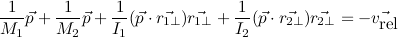## Monday, July 9, 2007

### Rigid Body Dynamics: Friction

Friction is tricky. It's generally formulated as a force, integrated over time. In my program as it stands now, only gravity is integrated; the rest of the simulation is handled via impulses between frames.

Numerical integration methods make simplifying assumptions about how forces will behave over the frame duration. The simplest is to assume forces won't change. For a uniform gravity field this is not a bad assumption. For friction, though, it's not a good assumption. Friction forces should never cause objects to reverse direction, which is entirely possible if you assume the friction doesn't change during a frame.

I am going to try and work friction into my impulse model. Brian Mirtich's thesis covers this in quite a lot of detail. Basically I will assume that friction doesn't change during a frame, but clamp it so that it never reverses object directions or adds energy.

This week I did a tiny step toward that. When a collision happens, I compute an impulse that cancels all relative motion at the collision point. This is equivalent to a completely inelastic collision (because motion in the normal direction is canceled) combined with infinite static friction (because all motion in the tangential direction is canceled). I decided to try this out because it's sort of the maximum friction possible; sliding friction and bouncing will involve reducing it somehow.

To come up with the proper impulse, I wrote an expression for how the relative velocity changes as a function of the (unknown) impulse, then set it equal to the negative of the relative velocity to be canceled:In the equation p is the unknown impulse. The r constants are the positions of the contact point with respect to the two bodies' centers of mass, but turned 90 degrees counterclockwise. I put the little T symbol on them to indicate that. Basically, these are the direction vectors for affecting the objects' rotational velocity. They scale up as the contact point gets further from the center of mass, since you get better leverage the further out you get. The M and I constants are the mass and rotational inertia tensor, respectively; in 2D they're both scalars.

This is a vector equation, so it works out to two scalar equations in two unknowns (the components of p). I used Cramer's Rule to solve the system of equations for the impulse.

With this friction, wheels roll, but they can never skid. I think I'll start to put together a vehicle this week. This will involve making some constraints to hold the wheels and the body together. I'll solve these the same way I solve nonpenetration constraints; by fixing up after integration.